# Precalculus : Express a Polynomial as a Product of Linear Factors

## Example Questions

← Previous 1

### Example Question #1 : Express A Polynomial As A Product Of Linear Factors

Express the polynomialas a product of linear factors.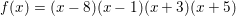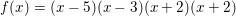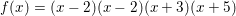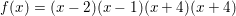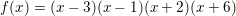Explanation:

We begin by attempting to find any rational roots using the Rational Root Theorem, which states that the possible rational roots are the positive or negative versions of the possible fractional combinations formed by placing a factor of the constant term in the numerator and a factor of the leading coefficient in the denominator.

That was a lot of wordage in one sentence, so let's break that down.  We begin with our polynomial.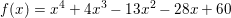The constant term is the term without a variable (just a plain number).  In our case the constant is 60.  What are the possible factors of 60?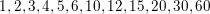The leading coefficient is the number in front of the largest power of the variable.  When the terms are listed in descending order (highest to lowest power), the leading coefficient is always the first number.  In our case the leading coefficient is hard to spot.  Since there is no number in front of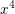, the coefficient is 1 by default.

This is nice because the only factor of 1 is well ... 1.

We then create all the possible fractions with a factor of the constant in the numerator and a factor of the leading coefficient in the denominator.  This actually isn't as bad as it could be since our only possible denominator is 1.  Any fraction with a denominator of 1 is just the numerator.  Therfore, our possible "fractions" are simplyHowever, we must consider the positive or negative versions of these, so our final list of possible rational roots is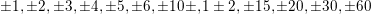Unfortunately, this is where the process (at least without the assitance of a graphing calculator) becomes less fun.  Using synthetic division, we must simply try each possible root until we have success. There's really no consistent rule to tell us where to start. Generally starting with the smaller whole numbers is best because the synthetic division is easier.  Therefore, we could begin withthen proceed to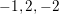, etc.

For the sake of keeping this explanation as short as possible, I am going to skip straight to 2, where we will first find success.

Therefore, 2 is a root.  However, it is always important to check to see if a root is in fact a double root (it works twice).  Therefore, let's try it one more time.

2 does in fact work twice and is thus a double root.  Since we only have three terms remainng, we can convert from synthetic back to an algebraic expression.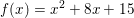We can then factor.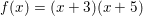Writing our root of 2 as an algebraic expression gives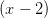.  Since we have double root, we need two of these.  Therfore, our final factored expression is.### Example Question #1 : Express A Polynomial As A Product Of Linear Factors

Which of the following represents the polynomial expression defined by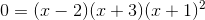in standard form?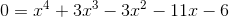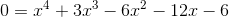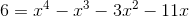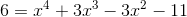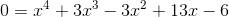Explanation:

Expansion and simplification give uswhich is properly expressed in standard form with the constant on the right. Below is a walkthrough of the expansion and simplification (including binomial and trinomial expansion techniques).

Remember to multiply each term in one polynomial with each term in the other polynomial.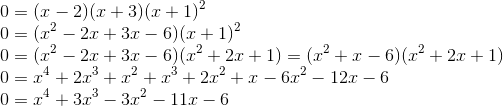### Example Question #3 : Express A Polynomial As A Product Of Linear Factors

Which of the following is equivalent to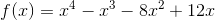?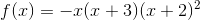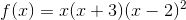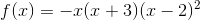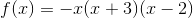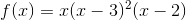Explanation:

To express this polynomial as a product of linear factors you have to find the zeros of the polynomial by the method of your choosing and then combine the linear expressions that yield those zeros.

Factoring will get you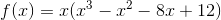, but then you are left to sort through the thrid degree polynomial. We can quickly synthetically divide the polynomial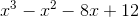by its potential roots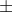factors of. So that's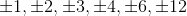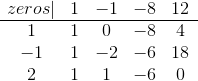We know thatis a zero and dividing the original polynomial byand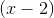gives us the polynomial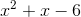. We can factor this or use the quadratic formula to give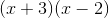.

This leaves us with four linear expressions that compose the polynomial: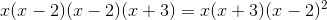### Example Question #2 : Express A Polynomial As A Product Of Linear Factors

A polyomial with leading term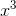has 5 and 7 as roots; 7 is a double root. What is this polynomial?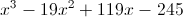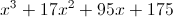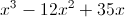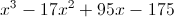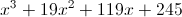Explanation:

Since 5 is a single root and 7 is a double root, and the degree of the polynomial is 3, the polynomial is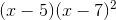. To put this in expanded form: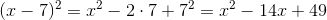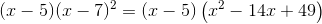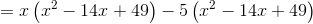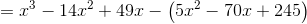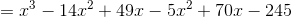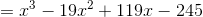### Example Question #1 : Express A Polynomial As A Product Of Linear Factors

Factorize the following polynomial expression completely to its linear factors: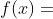### Example Question #2 : Express A Polynomial As A Product Of Linear Factors

Factorize the following expression completely to its linear factors:### Example Question #3 : Express A Polynomial As A Product Of Linear Factors

Explanation:

First, pull out the common factor of 2, and then factorize:### Example Question #4 : Express A Polynomial As A Product Of Linear Factors

Find the real zeros of the equation using factorization: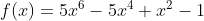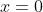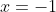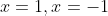There are no real zeros for this function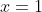### Example Question #1 : Express A Polynomial As A Product Of Linear Factors

Factorize the following expression completely to its linear factors: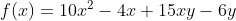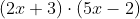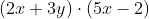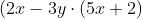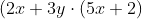Explanation:

Use the grouping method to factorize common terms: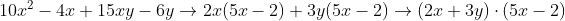### Example Question #2 : Express A Polynomial As A Product Of Linear Factors

Factorize the following expression completely to its linear factors: f(x)=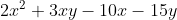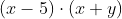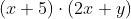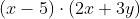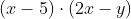Explanation:

Use grouping method to factorize common terms: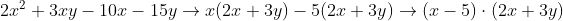← Previous 1

### All Precalculus Resources